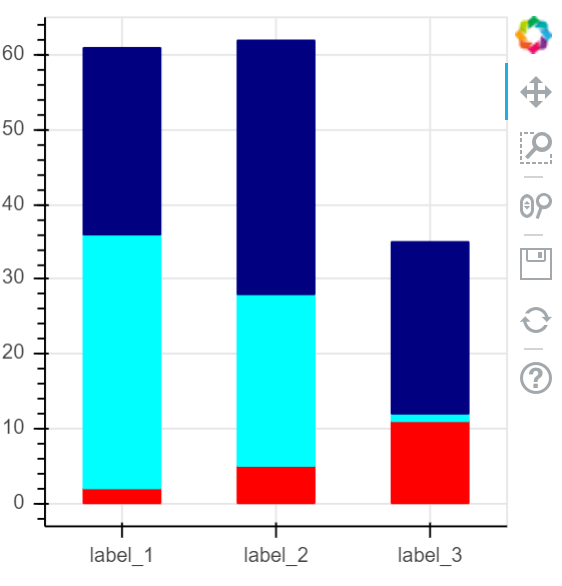# How can Bokeh library be used to visualize stacked bar charts in Python?

Bokeh is a Python package that helps in data visualization. It is an open source project. Bokeh renders its plot using HTML and JavaScript. This indicates that it is useful while working with web-based dashboards.

Bokeh converts the data source into a JSON file. This file is used as an input to BokehJS, which is a JavaScript library. This BokehJS is written in TypeScript that helps render visualization on modern browsers.

Matplotlib and Seaborn produce static plots, whereas Bokeh produces interactive plots. This means when the user interacts with these plots, they change accordingly.

Plots can be embedded as output of Flask or Django enabled web applications. Jupyter notebook can also be used to render these plots.

## Installation of Bokeh on Windows command prompt

pip3 install bokeh

Installation of Bokeh on Anaconda prompt

conda install bokeh

The ‘vbar_stack’ or ‘hbar_stack’ function can be used to generate stacked bar graphs.

## Example

from bokeh.plotting import figure, output_file, show
labs = ['label_1', 'label_2', 'label_3']
vals = ['val_1','val_2','val_3']
my_data = {'labs':labs,
'val_1':[2,5,11],
'val_2':[34,23,1],
'val_3':[25, 34, 23]
}
cols = ['red','cyan','navy']
fig = figure(x_range = labs, plot_width = 300, plot_height = 300)
fig.vbar_stack(vals, x = 'labs', source = my_data, color = cols,width = 0.5)
show(fig)

## Output## Explanation

• The required packages are imported, and aliased.

• The figure function is called along with plot width and height.

• The data is defined in lists.

• The ‘output_file’ function is called to mention the name of the html file that will be generated.

• The ‘vbar_stack’ function present in Bokeh is called, along with data.

• The ‘show’ function is used to display the plot.